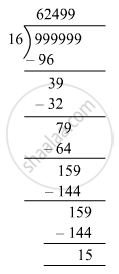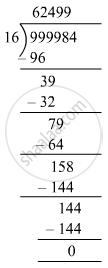# Find the largest 6-digit number divisible by 16. - Mathematics

Sum

Find the largest 6-digit number divisible by 16.

#### Solution

Largest six-digit number = 999999Remainder = 15

Largest six-digit number divisible by 16 = 999999 − 15 = 999984∴ 999984 is divisible by 16.

Concept: Properties of Whole Numbers
Is there an error in this question or solution?

#### APPEARS IN

RS Aggarwal Class 6 Mathematics
Chapter 3 Whole Numbers
Test Paper 3 | Q 6 | Page 59